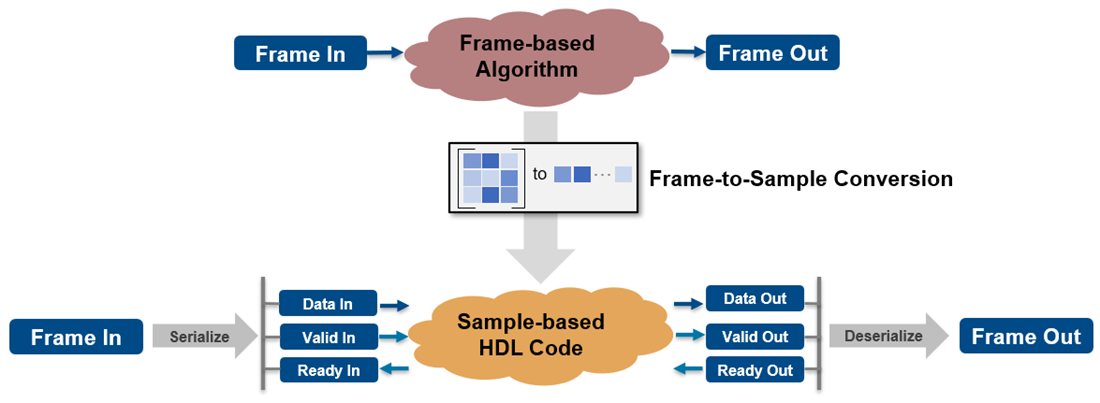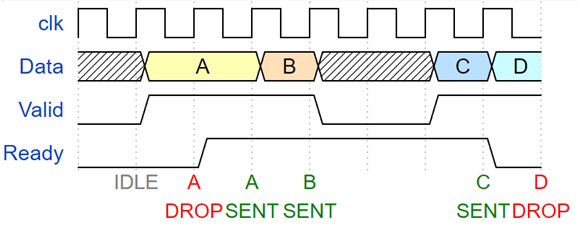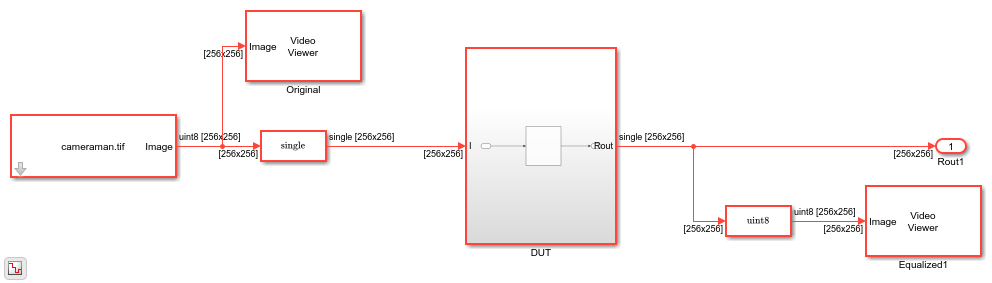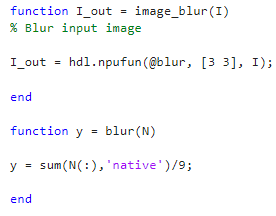## HDL Code Generation from Frame-Based Algorithms

Platforms that have limited I/O like FPGA or ASIC devices typically process large datasets as streaming pixels or samples. To deploy a frame-based model onto these devices, you must manually translate your algorithms to operate on streams of data. You can automate this process and generate HDL code from frame-based models or MATLAB® functions with matrix inputs by using the frame-to-sample optimization in HDL Coder™. This optimization converts frame-based vector or matrix inputs to smaller-sized samples or pixels for HDL code generation to target stream-based hardware and reduce the FPGA I/O needed to handle large input and output signals. You can optimize designs for hardware while reducing algorithm development time for various use cases in domains that have large inputs, such as image processing, digital signal processing, radar applications, and audio processing.

When you use the frame-to-sample optimization, HDL Coder generates hardware-ready HDL code from frame-based algorithms that has the necessary logic to store samples inside the DUT in line buffers, align streams, and balance data paths. You can use multiple modeling patterns, such as element-wise operations, neighborhood operations, and iterative and reduction operations, to author frame-based algorithms supported by the frame-to-sample optimization.

### Generating HDL Code from a Frame-based Algorithm

When you use the frame-to-sample conversion to generate HDL code from a frame-based algorithm, HDL Coder transforms your frame-based algorithm into synthesizable HDL code with sample-based logic that has valid and ready control signals and the logic to handle and align the data streams directly from the frame-based algorithm. You can use the frame-to-sample conversion with a Simulink® model or a MATLAB function.When you generate HDL code from a frame-based algorithm, the stream-based HDL code and generated model contain sample-based logic, which includes data signals, valid and ready control signals. This timing diagram maps the relationship between the Valid, Data, and Ready signals.A Valid signal indicates when data is available. The Ready signal indicates that the DUT can accept and process data. Transfer of data occurs only when both the Valid and Ready signals are high. This is represented by data packets A,B, and C in the image. When the Ready signal is low, the DUT cannot process more data. If you send a Valid and Data signal before the Ready signal assertion, the Data signal is dropped. You can de-assert the Valid signal and not send data while the Ready signal is high. If the Ready signal de-asserts and the Valid signal is high, the Data signal is dropped. This is represented by data packet D in the image.

You can create matrix or frame-based algorithms by using element-wise operations, neighborhood operations, and iterative and reduction operations supported for HDL code generation. You can create:

• Neighborhood operations by using the `hdl.npufun` function a in MATLAB function or the Neighborhood Processing Subsystem block in a Simulink model. You can use `hdl.npufun` in a MATLAB Function block in your DUT to apply neighborhood processing and element-wise operations to an incoming image or matrix, such as filtering with a kernel.

• Iterative operations using the `hdl.iteratorfun` function. For example, you can use `hdl.iteratorfun` in a MATLAB Function block in your DUT to loop over arrays to produce a single output to an incoming image or matrix for histogram equalization and to compute statistics such as `min` and `max`.

• Element-wise operations by using element-wise functions or blocks such as the Gain, Product, Sum, Subtract, and Divide blocks.

In vision or image processing, you can use these frame operations to model 2-D based algorithms, such as filtering, histogram creation, histogram equalization, and edge detection. In signal processing, you can calculate a moving average computation on a large input signal.

### Specify the Frame-to-Sample Conversion Optimization

You can generate HDL code with the frame-to-sample conversion optimization from a Simulink model or MATLAB function.

#### Specify the Frame-to-Sample Conversion Optimization from Simulink

To enable the frame-to-sample conversion optimization for a Simulink model:

1. Individually enable the HDL block property ConvertToSamples for Inport blocks on the DUT to convert the incoming vector or matrix input signal to samples by using the frame to sample conversion optimization. To specify an Inport block as a input signal for the frame-to-sample conversion, enter:

```hdlset_param("<path/to/Inport>", ConvertToSamples = "on") ```

2. Select the Enable frame to sample conversion parameter in the Configuration Parameters window. Use the frame-to-sample conversion parameters to apply frame-to-sample optimization options to your design. To enable frame-to-sample conversion on a Simulink model, on the command line, enter:

```hdlset_param("<model_name>", FrameToSampleConversion = "on") ```

3. If you design your frame-based algorithm using a MATLAB Function block, set the HDL block property Architecture of the MATLAB Function block to ```MATLAB Datapath```.

After generating HDL code, you can check the generated model and HDL code to see that each of the matrix inputs streamed contain a sample, valid, and ready bundle. You can use the validation model to compare the original frame-based model and the generated sample-based model. You can also deploy the generated HDL code to an FPGA using the IP core generation workflow. For an example, see Deploy a Frame-Based Model with AXI4-Stream Interfaces.

#### Specify Frame-to-Sample Conversion from MATLAB

To enable the frame-to-sample conversion optimization for a MATLAB function:

1. Open the MATLAB HDL Workflow Advisor. To get started with the MATLAB HDL Workflow Advisor, see Basic HDL Code Generation and FPGA Synthesis from MATLAB.

2. In the left pane, click the HDL Code Generation task. In the right pane, navigate to the Optimization tab and select Aggressive Dataflow Conversion.

3. Click the Frame to Sample Conversion tab and select Enable Frame to Sample Conversion. Use the frame-to-sample conversion parameters in this tab to apply optimization options to your design.

### Generate HDL Code from a Frame-Based Model Example

The Simulink model `hdlFrame_Blur_2D_MLFB` models a common image-processing frame-based blurring algorithm which uses a neighborhood processing pattern.

Open the model to view the frame-based blurring algorithm. The model applies an image-blurring kernel to the image `cameraman.tif`.

```open_system("hdlFrame_Blur_2D_MLFB"); set_param(gcs,'SimulationCommand','Update'); ```The DUT contains a MATLAB function called `image_blur` in the MATLAB Function block. The `image_blur` function calls the frame-to-sample supported function `hdl.npufun` that applies the blurring kernel in the `blur` function to the input image. For more information, see `hdl.npufun`.

```open_system("hdlFrame_Blur_2D_MLFB/DUT/MATLAB Function") ```Apply the frame-to-sample conversion optimization by setting the model configuration parameter `FrameToSampleConversion` to `on` from the command line.

```hdlset_param("hdlFrame_Blur_2D_MLFB", FrameToSampleConversion = "on"); ```

Specify which incoming frame-based signal to be convert to a sample-based signal by setting the HDL Inport block property `ConvertToSamples` of the input to `on`. In this example, set the `ConvertToSamples` property to `on` for the only Inport block to the `DUT` subsystem, `I`.

```hdlset_param("hdlFrame_Blur_2D_MLFB/DUT/I", ConvertToSamples = "on"); ```

To see the conversion from the frame-based model to the sample-based version, enable model generation. Generate HDL code and the generated model from the `DUT` subsystem.

```hdlset_param("hdlFrame_Blur_2D_MLFB", GenerateModel = "on"); makehdl('hdlFrame_Blur_2D_MLFB/DUT'); bdclose('hdlFrame_Blur_2D_MLFB/Original'); bdclose('hdlFrame_Blur_2D_MLFB/Equalized1'); ```
```### Generating HDL for 'hdlFrame_Blur_2D_MLFB/DUT'. ### Using the config set for model <a href="matlab:configset.showParameterGroup('hdlFrame_Blur_2D_MLFB', { 'HDL Code Generation' } )">hdlFrame_Blur_2D_MLFB</a> for HDL code generation parameters. ### Running HDL checks on the model 'hdlFrame_Blur_2D_MLFB'. ### Begin compilation of the model 'hdlFrame_Blur_2D_MLFB'... ### Working on the model 'hdlFrame_Blur_2D_MLFB'... ### The code generation and optimization options you have chosen have introduced additional pipeline delays. ### The delay balancing feature has automatically inserted matching delays for compensation. ### The DUT requires an initial pipeline setup latency. Each output port experiences these additional delays. ### Output port 1: 127 cycles. ### Output port 1: The first valid output of this port will be after an initial latency of 257 valid inputs. ### Output port 2: 127 cycles. ### Output port 2: The first valid output of this port will be after an initial latency of 257 valid inputs. ### Working on... <a href="matlab:configset.internal.open('hdlFrame_Blur_2D_MLFB', 'GenerateModel')">GenerateModel</a> ### Begin model generation 'gm_hdlFrame_Blur_2D_MLFB' .... ### Rendering DUT with optimization related changes (IO, Area, Pipelining)... ### Model generation complete. ### Delay absorption obstacles can be diagnosed by running this script: <a href="matlab:run('hdlsrc/hdlFrame_Blur_2D_MLFB/highlightDelayAbsorption')">hdlsrc/hdlFrame_Blur_2D_MLFB/highlightDelayAbsorption.m</a> ### To clear highlighting, click the following MATLAB script: <a href="matlab:run('hdlsrc/hdlFrame_Blur_2D_MLFB/clearhighlighting.m')">hdlsrc/hdlFrame_Blur_2D_MLFB/clearhighlighting.m</a> ### Begin VHDL Code Generation for 'hdlFrame_Blur_2D_MLFB'. ### MESSAGE: The design requires 65536 times faster clock with respect to the base rate = 1. ### Working on counterNetwork as hdlsrc/hdlFrame_Blur_2D_MLFB/counterNetwork.vhd. ### Working on NeighborhoodCreator_3x3/row3_col2 as hdlsrc/hdlFrame_Blur_2D_MLFB/row3_col2.vhd. ### Working on NeighborhoodCreator_3x3/row2_linebuffer/SimpleDualPortRAM_generic as hdlsrc/hdlFrame_Blur_2D_MLFB/SimpleDualPortRAM_generic.vhd. ### Working on NeighborhoodCreator_3x3/row2_linebuffer as hdlsrc/hdlFrame_Blur_2D_MLFB/row2_linebuffer.vhd. ### Working on NeighborhoodCreator_3x3 as hdlsrc/hdlFrame_Blur_2D_MLFB/NeighborhoodCreator_3x3.vhd. ### Working on boundaryCounters_3_3 as hdlsrc/hdlFrame_Blur_2D_MLFB/boundaryCounters_3_3.vhd. ### Working on BoundaryCheck_3x3 as hdlsrc/hdlFrame_Blur_2D_MLFB/BoundaryCheck_3x3.vhd. ### Working on I_NeighborhoodCreator as hdlsrc/hdlFrame_Blur_2D_MLFB/I_NeighborhoodCreator.vhd. ### Working on hdlFrame_Blur_2D_MLFB/DUT/MATLAB Function/blur/nfp_div_single as hdlsrc/hdlFrame_Blur_2D_MLFB/nfp_div_single.vhd. ### Working on hdlFrame_Blur_2D_MLFB/DUT/MATLAB Function/blur/nfp_add_single as hdlsrc/hdlFrame_Blur_2D_MLFB/nfp_add_single.vhd. ### Working on hdlFrame_Blur_2D_MLFB/DUT/MATLAB Function/blur as hdlsrc/hdlFrame_Blur_2D_MLFB/blur.vhd. ### Working on hdlFrame_Blur_2D_MLFB/DUT/MATLAB Function as hdlsrc/hdlFrame_Blur_2D_MLFB/MATLAB_Function.vhd. ### Working on hdlFrame_Blur_2D_MLFB/DUT/Input_FIFOs/I_FIFO as hdlsrc/hdlFrame_Blur_2D_MLFB/I_FIFO.vhd. ### Working on hdlFrame_Blur_2D_MLFB/DUT/Input_FIFOs as hdlsrc/hdlFrame_Blur_2D_MLFB/Input_FIFOs.vhd. ### Working on hdlFrame_Blur_2D_MLFB/DUT/Output_FIFOs/I_out_FIFO as hdlsrc/hdlFrame_Blur_2D_MLFB/I_out_FIFO.vhd. ### Working on hdlFrame_Blur_2D_MLFB/DUT/Output_FIFOs as hdlsrc/hdlFrame_Blur_2D_MLFB/Output_FIFOs.vhd. ### Working on hdlFrame_Blur_2D_MLFB/DUT as hdlsrc/hdlFrame_Blur_2D_MLFB/DUT.vhd. ### Generating package file hdlsrc/hdlFrame_Blur_2D_MLFB/DUT_pkg.vhd. ### Code Generation for 'hdlFrame_Blur_2D_MLFB' completed. ### Creating HDL Code Generation Check Report file:///tmp/Bdoc23b_2361005_1092267/tp19e60506/hdlcoder-ex36441221/hdlsrc/hdlFrame_Blur_2D_MLFB/DUT_report.html ### HDL check for 'hdlFrame_Blur_2D_MLFB' complete with 0 errors, 0 warnings, and 2 messages. ### HDL code generation complete. ```

Open the generated model, `gm_hdlFrame_Blur_2D_MLFB`. This model contains the sample-based version of the DUT and contains Valid, Ready, and Data signals.

```open_system('gm_hdlFrame_Blur_2D_MLFB'); Simulink.BlockDiagram.arrangeSystem('gm_hdlFrame_Blur_2D_MLFB'); ```Open the generated model DUT. There is significantly more hardware detail in the sampled-based generated model than in the original frame-based model. In the `DUT` subsystem, there is an input and output FIFO that handles and stores the data from the generated streaming matrix partition. The streaming matrix partition contains the blurring algorithm and other sample-based logic needed to deploy the algorithm to stream-based hardware.

```open_system('gm_hdlFrame_Blur_2D_MLFB/DUT'); ```If you run the generated model, notice that the time it takes to get the output image is longer than the original model. Converting a frame-based algorithm to a sample-based algorithm that streams pixels of the image one at a time requires more time to process each pixel than the frame-based version. The latency to process a valid output pixel for a valid input pixel from the original input frame depends on a few factors, such as size of the input image, the samples per cycle, and the algorithm. The output of the `makehdl` command displays the added latency in the MATLAB command window. In this example, the first valid output is accessible after an initial latency of 257 valid inputs.

The sampling rate, latency, and image size determine the simulation stop time needed for the generated model. To generate the blurred output image from the sample-based algorithm in the generated model, the original and subsequent generated model have stop times of 2 seconds, which is the time needed to display the entire output image after latency is introduced.

### Hardware Considerations

Prior to generating HDL code from frame-based algorithms for hardware deployment:

• Check that the target hardware should have enough memory to store frames in memory before and after the stream-based algorithm is applied. Sample-based algorithms require more memory, but use significantly less I/O.

• Ensure your design can handle some latency. Sample-based algorithms add latency and delay depending on your algorithm, design, and input data size.

### Supported Blocks and Operations

Frame-to-sample conversion supports these blocks and operations:

• MATLAB Function block with the HDL block property Architecture set to `MATLAB Datapath`. In the MATLAB function, you can use:

• Frame-to-sample-focused functions, such as `hdl.npufun` and `hdl.iteratorfun`.

• Element-wise operations between streamed data.

• Operations between a scalar and streamed data.

• Neighborhood Processing Subsystem block.

• Element-wise operations. For example, you can use blocks that support element-wise operations such as the Gain, Product, Sum, Subtract, and Divide blocks.

• Square matrix product operations. For example, you can use the Matrix Multiply block with square input matrices.

• 3-D matrices.

• Constant inputs or sources.

• Saturation.

• Trigonometric operations.

• Bit-wise operations, such as bit-slice, bit-and, and bit-or.

• Comparison operations.

• Bias block.

• Abs block.

• Complex to Real-Imag block and Real-Imag to Complex block.

• Sqrt block.

• Data type conversion block.

• Switch block.

### Limitations

Frame-to-sample conversion does not support:

• Column vectors.

• Persistent variables.

• Non-square matrix product operations.

• Matrix operations on streamed data. For example, frame-to-sample conversion in Simulink does not support using the Selector, Assignment, or Reshape block on streamed data.

• Sum of elements operations.

• Product of elements operations.

• MATLAB System Objects such as `hdl.RAM`.

• For Each Subsystems.

• Delay blocks.

• Blocks that contain design delays.

• `Bus` objects as a datatype.

• IP core generation from a MATLAB function.